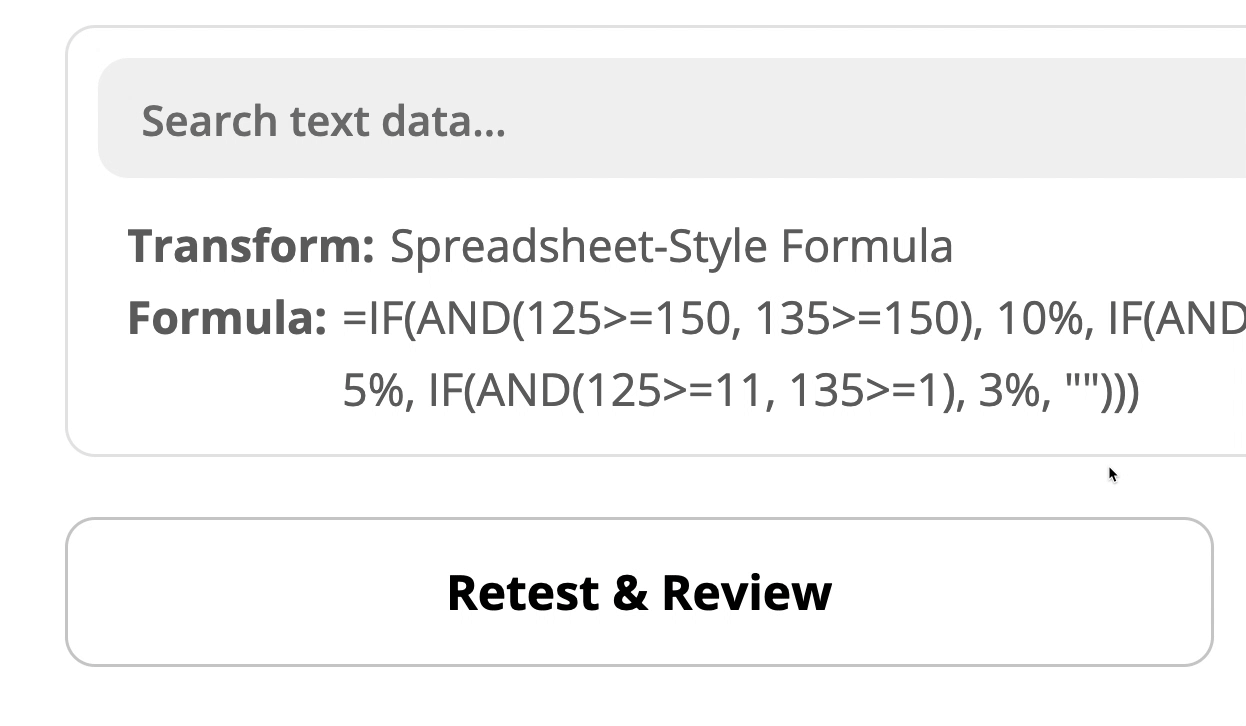# Formatter Features: a brief introduction to spreadsheet-style formulasUserlevel 7+11
• nicksimard• Community Manager
• 1952 replies

Welcome to the very first post in our new article series, Formatter Features! In this article we’re going to cover the basics of the spreadsheet-style formulas transform, in preparation for the next 3 pieces, which will dig into some more use cases and examples.

Definition: When we say “transform” we’re referring to the various functions within the Formatter by Zapier app. For example, spreadsheet-style formulas, format phone number, remove HTML tags, etc.

### So, What Are Spreadsheet-Style Formulas?

If you’re familiar with using formulas in popular spreadsheet apps like Excel, Numbers and Google Sheets, you can use spreadsheet-style formulas in Zaps with the same syntax.

It can be used with numbers or with text. That means you can find it here…

and also here:

### What Types of Things Can You Do With Them?

We have an extensive list here, in our help docs. Take a look at some of the things that are possible:

A few of the more popular functions

This is just a handful of the many functions that are available.

• IF(condition, true_value, [false_value]): Returns the true_value if the condition is TRUE or false_value if the condition is FALSE
• ISBLANK(value): Returns TRUE if a value is blank (empty); Otherwise, returns FALSE
• OR(condition, [condition, …]): Returns TRUE if any of the conditions evaluate to TRUE, otherwise FALSE
• RANDBETWEEN(min, max): Returns a random number between two integers
• Multiplication Operator: A * B multiplies A by B
• Division Operator: A / B divides A by B
• Subtraction Operator: A - B subtracts B from A
• Equal Condition: A = B is TRUE when A is equal to B
• Not Equal Condition: A <> B is TRUE when A is not equal to B

### Are They Hard to Use?

Not all formulas are created equally, in terms of difficulty level. Some are very basic and easy to understand, while others are conceptually challenging and require very specific syntax that may not be logical. After all, reading through some of these formulas you’ll notice that it’s not always obvious in which order you would place the data.

Things get tricky when you start to nest formulas

Here’s an example where I’ve got multiple nested IF/AND statements, to check whether `number one` AND `number two` are both greater than a given value. It goes through each option until it finds the first one that matches:

If I were to miss a single parenthesis, comma, or quotation the entire thing would break. Or, if one of the mapped values did not come through for some reason.

I removed the very last closing parenthesis and check out what happens:### What’s Next?

In the next article (and the two after it) we’ll be exploring some more detailed examples of how to use spreadsheet-style formulas to make your Zaps more powerful and efficient :)